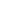| lesson material | material summary | questions and answers | definitions | types and examples | other information | materi pelajaran | ringkasan materi | pertanyaan dan jawaban | definisi | jenis-jenis dan contoh-contoh | informasi lainnya |

# Learning Javascript: Understanding Array Data Structures in Javascript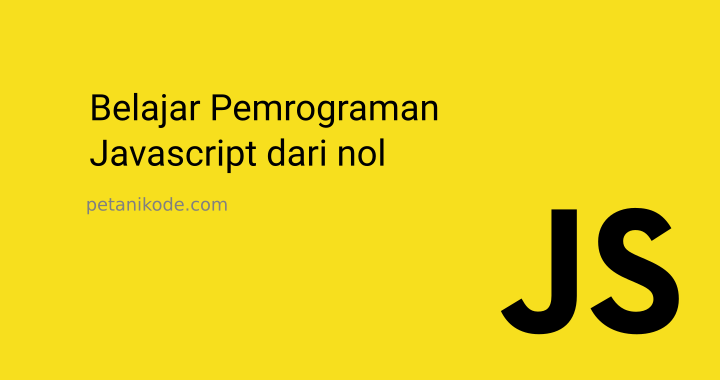Imagine now that we are making a web application, then want to display a list of product names.
We can make it like this:
``````var produk1 = "Modem";
var produk2 = "Hardisk";
var produk3 = "Flashdisk";

document.write(`\${produk1}<br>`);
document.write(`\${produk2}<br>`);
document.write(`\${produk3}<br>`);
``````
Can it be like this?
It is allowed. But it is less effective.
Why?
What if there were 100 products, would we make 100 variables and do `echo`100x?
I'm tired.
Therefore, we must use Arrays.

## What is an Array?

Before we discuss Array, we discuss first what is data structure?
Data structure is the methods or methods used to store data in computer memory.
One data structure that is often used in programming is Array .
An array is a data structure that is used to store a set of data in one place.
Each data in an array has an index, so we will easily process it.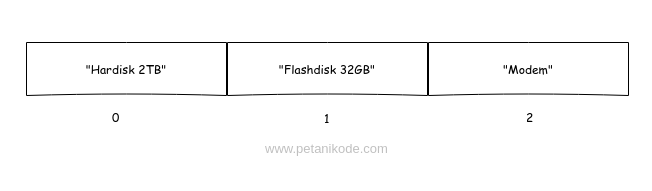The array index always starts from zero ( `0`).
In the data structure theory ...
... The size of the array will depend on how much data is stored in it.

## How to make an array in Javascript

In javascript, we can create arrays with square brackets ( `[...]`).
Example:
``````var products = [];
``````
Then the variable `products`will contain an empty array.
We can fill in the data into the array, then each data is separated by a comma ( `,`).
Example:
``````var products = ["Flashdisk", "SDD", "Monitor"];
``````
Anyway, because JavaScript is a dynamic typing programming language ...
... then we can store and mix anything in the array.
Example:
``````var myData = [12, 2.1, true, 'C', "Petanikode"];
``````

## How to retrieve data from an array

The array will store a set of data and give it an index number to make it easily accessible.
The array index is always monitored from zero `0`.
Suppose we have an array like this:
``````var makanan = ["Nasi Goreng", "Mie Ayam", "Mie Gelas"];
``````
How do we take value `"Mie Ayam"`?
``````makanan //-> "Mie Ayam"
``````
Why not `2`?
Remember: array indices always start from zero.
Let me be clearer, let's try it in the program:
``````<!DOCTYPE html>
<html lang="en">
<title>Mengambil data dari array</title>
<body>
<script>
// membuat array
var products = ["Senter", "Radio", "Antena", "Obeng"];

document.write(products);
</script>
</body>
</html>
``````
The result: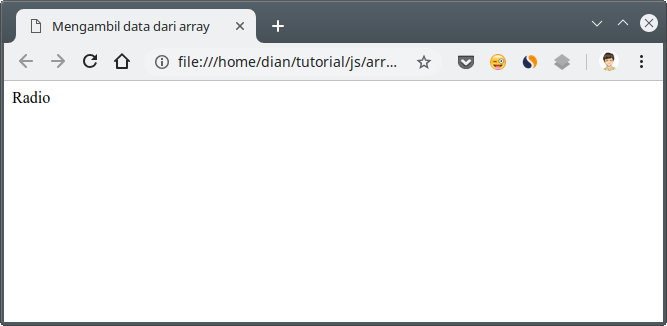## Print Array contents with Repetition

We can print all the contents of an array one by one like this:
``````document.write(products);
document.write(products);
document.write(products);
document.write(products);
document.write(products);
``````
What if later the contents of the array are there `100`?
Of course we won't want to write `100` lines of code to print arrays.
The solution: Use looping .
Let's look at an example:
``````<!DOCTYPE html>
<html lang="en">
<title>Array dan perulangan</title>
<body>
<script>
// membuat array
var products = ["Senter", "Radio", "Antena", "Obeng"];

document.write("<h3>Daftar Produk:</h3>");
document.write("<ol>");
// menggunakan perulangan untuk mencetak semua isi array
for(let i = 0; i < products.length; i++){
document.write(`<li>\${ products[i] }</li>`);
}
document.write("</ol>");
</script>
</body>
</html>
``````
The result: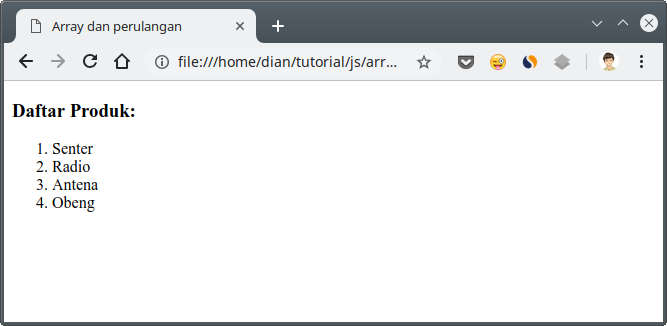Pay attention ...
In the example above, we use properties `length`to take the length of the array.
We have `4`data in the array `products`, then the property `length`will be worth `4`.
Then we use this property to limit the number of loops in for .
``````for(let i = 0; i < products.length; i++){
document.write(`<li>\${ products[i] }</li>`);
}
``````
... and in block for, we print product contents with indices that refer to variables `i`.
Another way:
We can use looping method `forEach()`.
Example:
``````<!DOCTYPE html>
<html lang="en">
<title>Array dan perulangan</title>
<body>
<script>
// membuat array
var products = ["Senter", "Radio", "Antena", "Obeng"];

document.write("<h3>Daftar Produk:</h3>");
document.write("<ol>");
// menggunakan perulangan untuk mencetak semua isi array
products.forEach((data) => {
document.write(`<li>\${data}</li>`);
});
document.write("</ol>");
</script>
</body>
</html>
``````
The result will be the same as above.## How to Add Data to Arrays

There are two ways you can add data to an array:
1. Fill in using indexes;
2. Fill in using method `push()`.
Fill in the index with this intention ...
Suppose we have an array with the contents as follows:
``````var buah = ["Apel", "Jeruk", "Manggis"];
``````
There are three data in an array `buah` with an index:
• `0``"Apel"`
• `1``"Jeruk"`
• `2``"Manggis"`
We want to add more data to the index `3`, so we can do it like this:
``````buah = "Semangka";
``````
An array `buah`will contain `4`data.
Let's try the Javascript console .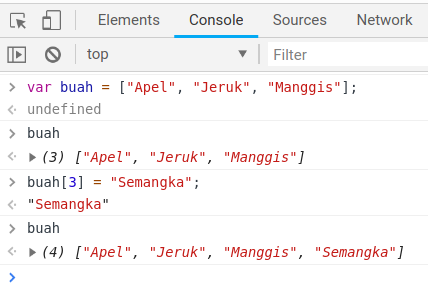Right ...
`"Semangka"`we have added it to the array `buah`.
But the disadvantages of this method are:
We must know the amount of data or the length of the array, then we can add.
If we enter an arbitrary index number, then what will happen is that the data in the index will be overwritten.
Then what is the solution?
We use the method `push()`.
We don't need to know how long the array is, because the method `push()`will add data to the array from the tail or back.
Example:
``````<!DOCTYPE html>
<html lang="en">
<title>Mengisi data ke array</title>
<body>
<script>
// membuat array
var products = ["Senter", "Radio", "Antena", "Obeng"];

// menambahkan tv ke dalam array products
products.push("Televisi");

// menapilkan isi array
document.write(products);
</script>
</body>
</html>
``````
Then the result: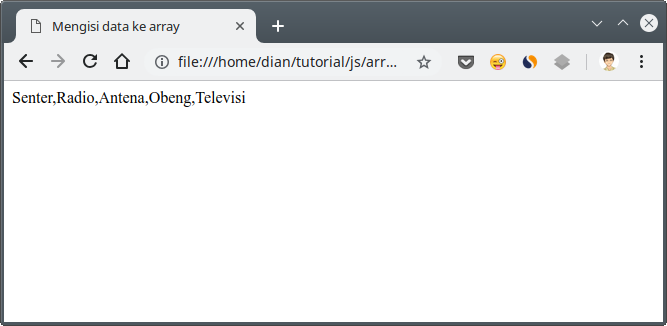We can also add multiple data at once in this way:
``````products.push("Alarm", "Gemobok", "Paku");
``````

## How to delete array data

Just like adding data to an array, deleting data also has two ways:
1. Use `delete`;
2. Using method `pop()`.
Example:
``````delete buah;
``````
We can delete data with certain index numbers with `delete`Whereas it `pop()`will remove from behind.
Short of `delete`, it will create empty space in the array.
Experiments in the console :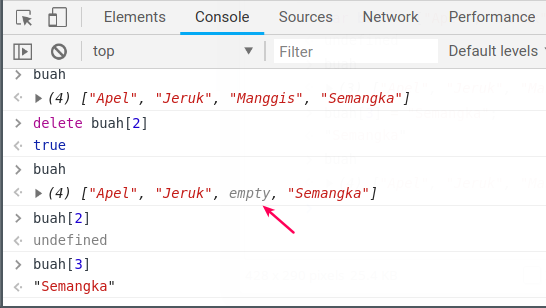Of course this is not good ...
... because the array will still have length `4`.
The second method uses the method `pop()`, the opposite of the method `push()`.
The method `pop()`will delete the array at the back.
We can see the array in JavaScript as a stack , which has LILO (Last in Last out) properties .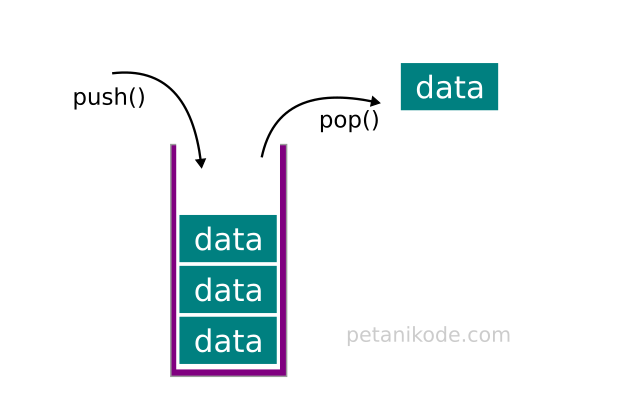Let's try it in the console .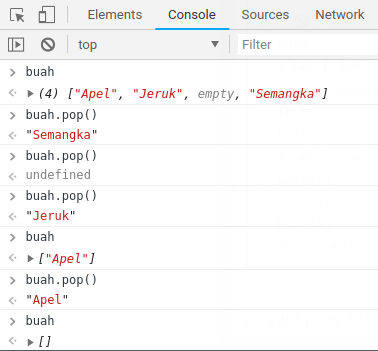We call the method `pop()`as many `4`times, then the array will be empty `[]`Because the contents are just `4`that.
The method `pop()`will return the item value or data deleted from the array.

### Deleting Data from Front

We can also delete data from the front using the method `shift()`.
Example:
``````var bunga = ["Mawar", "Melati", "Anggrek", "Sakura"];

// hapus data dari depan
bunga.shift();
``````
Then the deleted data is `"Mawar"`.
Experiment on conosole:

### Deleting Data on a Specific Index

If we want to delete data at certain inteks, then the function or method used is `splice()`.
This function has two parameters that must be given:
``````array.splice(<indeks>, <total>);
``````
Information:
• `<indeks>` is the index of the data in the array to be deleted;
• `<total>` is the amount of data to be deleted from that index.
Usually we give a total value with a value `1`to only delete one data.
Example:
``````var bunga = ["Mawar", "Melati", "Anggrek", "Sakura"];

// hapus Anggrek
bunga.splice(2, 1);
``````
Experiment on the console :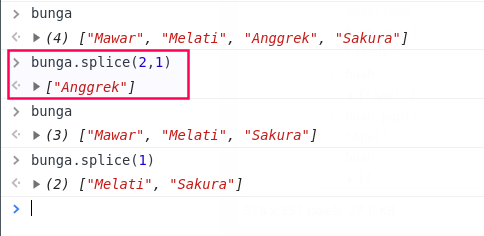In the experiment above, if we do not fill in the `<total>`data to be deleted, then all data from the selected index will be deleted.

## Change Array contents

To change the contents of an array, we can refill it like this:
``````var bahasa = ["Javascript", "Kotlin", "Java", "PHP", "Python"];
bahasa = "C++";
``````
Then it `"Kotlin"`will be replaced with `"C++"`.
Experiment on the console :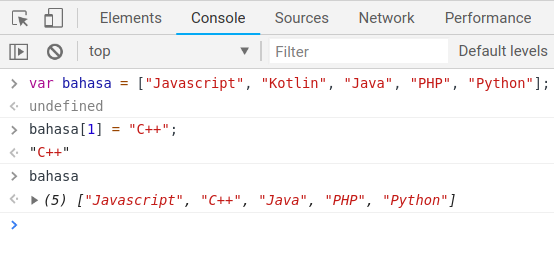It's easy not ...

## Method-mothod Array

In addition to the methods or functions we have tried above, there are several methods in Array that we need to know.

### 1. Method `filter()`

The method `filter()`functions to filter data from arrays.
The parameters that must be given to the method are the `filter()`same as the method `forEach()`, namely: a callback function .
Example:
``````const angka = [1, 2, 3, 4, 5, 6, 7, 8, 9];

// Kita ambil data yang hanya habis dibagi dua saja
const filteredArray = angka.filter((item) => {return item % 2 === 0});

console.log(filteredArray) // -> [2, 4, 6, 8]
``````
In the example above, we give arrow function as a callback function that will filter arrays.
Actually we can make it simpler like this:
``````const filteredArray = angka.filter(item => item % 2 === 0);
``````

### 2. Method `includes()`

This method functions to check whether a data is in an array or not. Usually used to do a search to make sure the data is already in the array.
Example:
``````var tanaman = ["Padi", "Kacang", "Jagung", "Kedelai"];

// apakah kacang sudah ada di dalam array tanaman?

``````

### 3. Method `sort()`

The method `sort()`functions to sort data in an array.
Example:
``````var alfabet = ['a','f','z','e','r','g'];
var angka = [3,1,2,6,8,5];

console.log(alfabet.sort()); //->  ["a", "e", "f", "g", "r", "z"]
console.log(angka.sort()); // -> [1, 2, 3, 4, 5, 6, 7, 8, 9]``````

Reference: https://www.petanikode.com/javascript-array/
##### 0 Komentar untuk "Learning Javascript: Understanding Array Data Structures in Javascript"

Silahkan berkomentar sesuai artikel

Template By Kunci Dunia